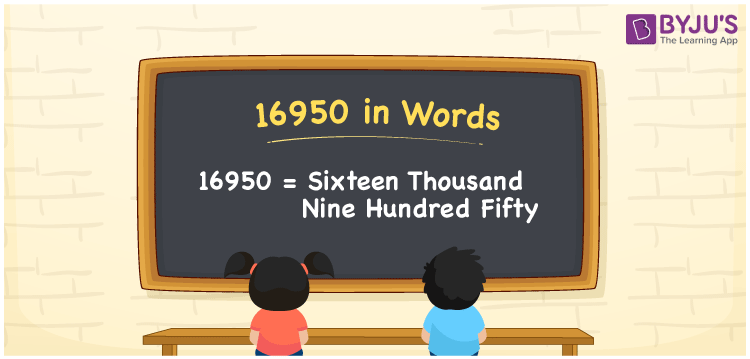# 16950 in Words

16950 in words is written as Sixteen Thousand Nine Hundred Fifty. For example, on a cheque, Rs. 16950 is given by “Rupees Sixteen Thousand Nine Hundred Fifty only”. The number 16950 is a cardinal number that is used to denote the quantity or value of something. 16950 can be used to represent the population, price of products, currency etc. Let us learn how to write the number 16950 in word form using a place value method.

 16950 in Words Sixteen Thousand Nine Hundred Fifty Sixteen Thousand Nine Hundred Fifty in numerical form 16950

## 16950 in English Words

In Mathematics, students are taught to write the numbers in words using the English alphabet. Hence, the number 16950 in English words can be expressed as Sixteen Thousand Nine Hundred Forty.## How to Write 16950 in Words?

We use a place value chart to represent the number in words with ease since the place value chart helps us to write the numerical name effortlessly. 16950 is a five-digit number. So, let us prepare a table of 5 columns. Check the place value chart for the number 16950 from the table provided below.

 Ten-Thousands Thousands Hundreds Tens Ones 1 6 9 5 0

Hence, we can write the expanded form as:

1 x Ten Thousand + 6 x Thousand + 9 x Hundred + 5 x Ten + 0 x One

= 1 x 10000 + 6 x 1000 + 9 x 100 + 5 x 10 + 0 x 1

= 10000 + 6000 + 900 + 50 + 0

= 10000 + 6000 + 900 + 50

= 16950

= Sixteen Thousand Nine Hundred Fifty

Therefore, 16950 in words is written as Sixteen Thousand Nine Hundred Fifty

Interesting way of writing 16950 in words

1 = One

16 = Sixteen

169 = One Hundred and Sixty-Nine

1695 = One Thousand Six Hundred Ninety-Five

16950 = Sixteen Thousand Nine Hundred Fifty

Thus, the word form of the number 16950 is Sixteen Thousand Nine Hundred Fifty

16950 is a natural number that is the successor of 16949 and the predecessor of 16951

• 16950 in words – Sixteen Thousand Nine Hundred Fifty
• Is 16950 an odd number? – No
• Is 16950 an even number? – Yes
• Is 16950 a perfect square number? – No
• Is 16950 a perfect cube number? – No
• Is 16950 a prime number? – No
• Is 16950 a composite number? – Yes

## Frequently Asked Questions on 16950 in Words

Q1

### How do you write 16950 in words?

We can write 16950 in words as Sixteen Thousand Nine Hundred Fifty.
Q2

### Simplify 15000 + 1950, and express in words.

Simplifying 15000 + 1950, we get 16950. Thus, 16950 in words is Sixteen Thousand Nine Hundred Fifty.
Q3

### How to spell 16950 in English words?

In English, the number 16950 is spelt as Sixteen Thousand Nine Hundred Fifty.